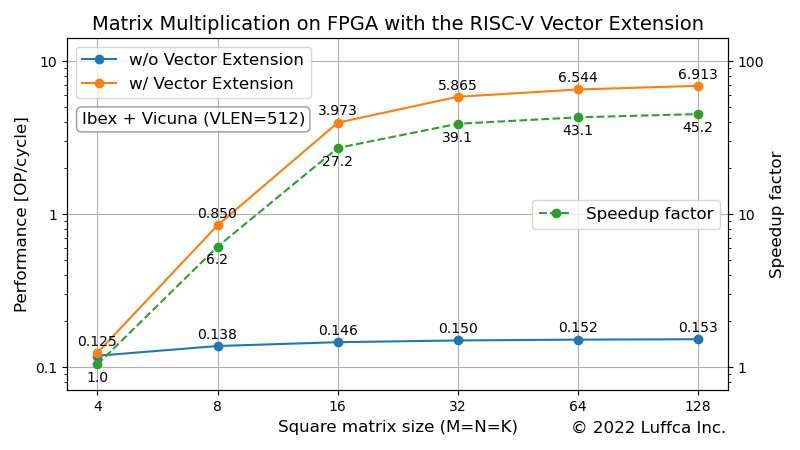# Matrix Multiplication on FPGA with the RISC-V Vector ExtensionWe have implemented Vicuna, an implementation of the RISC-V Vector Extension, on an FPGA board and evaluated the performance of the matrix multiplication kernel.

## Vicuna

Vicuna is a 32-bit integer vector coprocessor written in SystemVerilog. More precisely, Vicuna complies with the `Zve32x` extension that supports vector element widths of 8, 16, and 32 bits and does not require 64-bit elements or floating point support. However, at the time of writing, the divide instructions are missing.

Since Vicuna is a co-processor, it requires a main processor, Ibex or CV32E40X.

## FPGA with Vicuna

This time, we have created gateware for Digilent’s FPGA board Nexys Video.

The main specifications of the gateware for Nexys Video are as follows.

• Processor
• Main processor: Ibex
• Co-processor: Vicuna
• VLEN (bit length of vector register): 512-bit
• Multiplier bit length: 256-bit
• ISA: RV32IMCV
• Operating frequency: 100 MHz
• SRAM: 256 KiB
• UART: 1 ch

## Matrix Multiplication on FPGA with Vicuna

We used the code below as a reference kernel for matrix multiplication.

```// C = AB with A = [M x K], B = [K x N], C = [M x N]
void imatmul_ref(const int M, const int N, const int K, const int32_t* A,
const int32_t* B, int32_t* C) {
int i, j, k;
int32_t sum;
for (i = 0; i < M; ++i) {
for (j = 0; j < N; ++j) {
sum = 0;
for (k = 0; k < K; ++k) {
sum += A[i * K + k] * B[k * N + j];
}
C[i * N + j] = sum;
}
}
}
```

As in other articles we had the elements of matrices A and B set to `int8_t`, but changed them to `int32_t` because Vicuna’s `vsext.vf2` was giving incorrect results. Upon investigation, an issue was raised on GitHub.

The featured image above shows Vicuna’s performance.

When the square matrix size (M=N=K) is 32, 64 and 128, the performance [OP/cycle] of the matrix multiplication kernel based on the RISC-V Vector Extension is 5.865, 6.544 and 6.913 respectively. Compared to the reference kernel, we get a speedup of 39-45x.

## Summary

We have implemented Vicuna, which complies with the RISC-V Vector Extension `Zve32x`, on an FPGA board and evaluated the performance of the matrix multiplication kernel.

Previous article

Next article